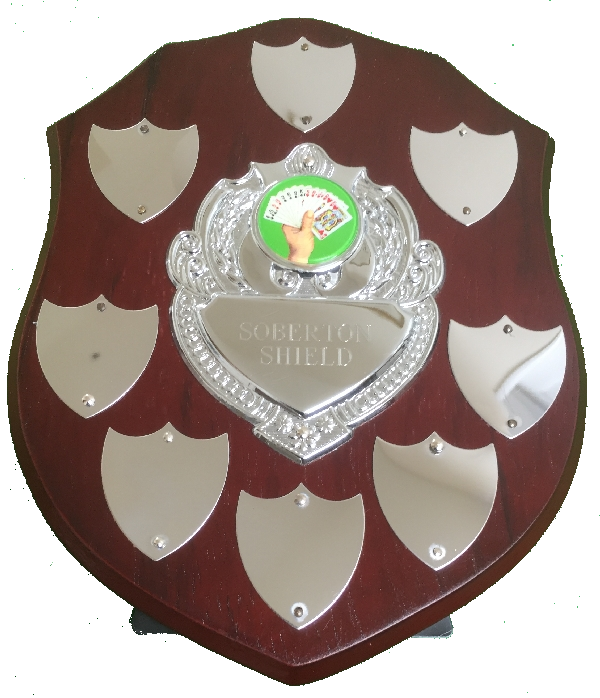# Soberton Shield Results - 2017

Team Jan 6 Feb 3 Mar 3 Apr 7 Oct 6 Nov 3 Total
Forrest Gumps (1) 28 -18 = 10 30 -4 = 26 32 -21 = 11 48 -23 = 25 61 +4 = 65 54 -13 = 41 178
Bridgemates (2) -12 -3 = -15 -2 +1 = -1 -6 +5 = -1 22 +7 = 29 -19 -11 = -30 -11 +14 = 3 -15
Six Hearts (3) 36 -15 = 21 40 -20 = 20 -10 -12 = -22 3 -17 = -14 33 -13 = 20 81 -5 = 76 101
Overcallers (4) 82 -13 = 69 68 -19 = 49 25 -9 = 16 48 -14 = 34 53 -11 = 42 13 -6 = 7 217
The Acolites (5) 22 -8 = 14 -78 -8 = -86 65 -14 = 51 17 -14 = 3 13 -6 = 7 66 -8 = 58 47
A Bridge Too Far (6) -53 +19 = -34 -66 +24 = -42 -33 +21 = -12 -89 +21 = -68 -15 +18 = 3 -98 +15 = -83 -236
Waltham Wanderers (7) -47 +6 = -41 40 -4 = 36 -62 +3 = -59 6 +10 = 16 -27 -6 = -33 -2 -9 = -11 -92
The Grand Slammers (8) -18 +19 = 1 14 +16 = 30 -2 +17 = 15 -4 +15 = 11 -57 +12 = -45 -57 -4 = -61 -49
Ruffstuff (9) -33 +13 = -20 -49 +14 = -35 -9 +10 = 1 -51 +15 = -36 -42 +12 = -30 -46 +15 = -31 -151
Total IMPs 168 - 163 = 5 192 - 195 = -3 122 - 122 = 0 144 - 144 = 0 160 - 160 = 0 214 - 214 = 0

Under each date for each team is a sum which represents 'IMPs' + 'Handicap' = 'Total'.
The row 'Total IMPs' shows the 'sum of plus scores' - 'the sum of minus scores' = X where X should be zero.

Click on a table heading to sort the column under it.